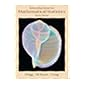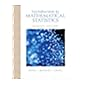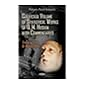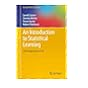Dr. S. R. Lasker Library Online Catalogue

Normal view

# Introduction to mathematical statistics / Robert V. Hogg, Allen T. Craig.

Material type:TextLanguage: English Publication details: c1978. Edition: 4th edDescription: x, 438 p. : ill. ; 23 cmISBN: 0023557109; 9780023557101; 0029789907Subject(s): Mathematical statistics | StatisticsDDC classification: 519 LOC classification: QA276 | .H59 1978Online resources: WorldCat details
Contents:
TOC Distributions of random variables -- Conditional probability and stochastic independence -- Some special distributions -- Distributions of functions of random variables -- Limiting distributions -- Estimation -- Statistical hypotheses -- Other statistical tests -- Nonparametric methods -- Sufficient statistics -- Further topics in statistical inference -- Further normal distribution theory.
Tags from this library: No tags from this library for this title.
Star ratingsAverage rating: 0.0 (0 votes)
Holdings
Item type Current library Collection Call number Copy number Status Date due Barcode Item holdsText
Reserve Section
Non-fiction 519.5 HOT 1978 (Browse shelf(Opens below)) C-1 Not For Loan 984
Total holds: 0
##### Browsing EWU Library shelves, Shelving location: Reserve Section Close shelf browser (Hides shelf browser)No cover image available 519.5 HOI 2005 Introduction to mathematical statistics / 519.5 HOI 2005 Introduction to mathematical statistics / 519.5 HOI 2014 Introduction to mathematical statistics / 519.5 HOT 1978 Introduction to mathematical statistics / 519.5 HUC 2013 Collected volume of statistical works of Q.M. Husain with commentaries / 519.5 INT 2017 An introduction to statistical learning : 519.5 ISI 2001 Introduction to statistics and probability /

Online version:
Hogg, Robert V.
Introduction to mathematical statistics.
New York : Macmillan, c1978
(OCoLC)647656341

Includes bibliographical references and index.

TOC Distributions of random variables --
Conditional probability and stochastic independence --
Some special distributions --
Distributions of functions of random variables --
Limiting distributions --
Estimation --
Statistical hypotheses --
Other statistical tests --
Nonparametric methods --
Sufficient statistics --
Further topics in statistical inference --
Further normal distribution theory.

AS

Saifun Momota

There are no comments on this title.